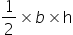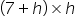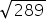Maths-
General
Easy

Question

# The difference between the sides of the right angles triangle containing the right angle is 7 cm, and its area is 60 cm2 . Find the perimeter of the triangle.Hint:

## The correct answer is: 40 cm

### It is given that difference between perpendicular and base = 7i.e. b – h = 7  ⇒  b = 7 + hNow, Area of triangle = 60 cm2= 60= 120h2 + 7h – 120 = 0h2 +15h – 8h – 120 = 0h(h + 15)-8(h + 15) = 0(h + 15)(h – 8) = 0h = 8 , - 15Since, perpendicular is always positive so h = 8Base, B = 7 + 8 = 15Using Pythagoras theorem ,H2 = B2 + P2H2 = 152 + 82 = 225 + 64 = 289H == 17 cmPerimeter of triangle = Sum of all sides= 17 + 8 + 15 = 40 cm#### With Turito Foundation.#### Get an Expert Advice From Turito.